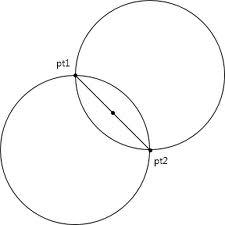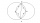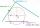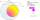# Common chord

Two circles with radius 17 cm and 20 cm are intersect at two points. Its common chord is long 27 cm. What is the distance of the centers of these circles?

Correct result:

l =  25.1 cm

#### Solution:We would be pleased if you find an error in the word problem, spelling mistakes, or inaccuracies and send it to us. Thank you!Tips to related online calculators
Pythagorean theorem is the base for the right triangle calculator.

#### You need to know the following knowledge to solve this word math problem:

We encourage you to watch this tutorial video on this math problem:

## Next similar math problems:

• CirclesIn the circle with a radius 7.5 cm are constructed two parallel chord whose lengths are 9 cm and 12 cm. Calculate the distance of these chords (if there are two possible solutions write both).
• Two chordsIn a circle with radius r = 26 cm two parallel chords are drawn. One chord has a length t1 = 48 cm and the second has a length t2 = 20 cm, with the center lying between them. Calculate the distance of two chords.
• ChordIn a circle with radius r=60 cm is chord 4× longer than its distance from the center. What is the length of the chord?
• Two circlesTwo circles with the same radius r = 1 are given. The center of the second circle lies on the circumference of the first. What is the area of a square inscribed in the intersection of given circles?
• Eq triangle minus arcsIn an equilateral triangle with a 2cm side, the arcs of three circles are drawn from the centers at the vertices and radii 1cm. Calculate the content of the shaded part - a formation that makes up the difference between the triangle area and circular cuts
• Two parallel chordsIn a circle 70 cm in diameter, two parallel chords are drawn so that the center of the circle lies between the chords. Calculate the distance of these chords if one of them is 42 cm long and the second 56 cm.
• Concentric circles and chordIn a circle with a diameter d = 10 cm, a chord with a length of 6 cm is constructed. What radius have the concentric circle while touch this chord?
• Sphere cutsAt what distance from the center intersects sphere with radius R = 56 plane, if the cut area and area of the main sphere circle is in ratio 1/2.
• Concentric circlesIn the circle with diameter 19 cm is constructed chord 9 cm long. Calculate the radius of a concentric circle that touches this chord.
• TangentWhat distance is the tangent t of the circle (S, 4 cm) and the chord of this circle, which is 6 cm long and parallel to the tangent t?
• Two chordsCalculate the length of chord AB and perpendicular chord BC to circle if AB is 4 cm from the center of the circle and BC 8 cm from the center of the circle.
• Chord ABWhat is the length of the chord AB if its distance from the center S of the circle k(S, 92 cm) is 10 cm?
• Circle chordCalculate the length of the chord of the circle with radius r = 10 cm, length of which is equal to the distance from the center of the circle.
• Chors centersThe circle with a diameter 17 cm, upper chord /CD/ = 10.2 cm and bottom chord /EF/ = 7.5 cm. The midpoints of the chords H, G is that /EH/ = 1/2 /EF/ and /CG/ = 1/2 /CD/. Determine the distance between the G and H, if CD II EF (parallel).
• TangentsTo circle with a radius of 41 cm from the point R guided two tangents. The distance of both points of contact is 16 cm. Calculate the distance from point R and circle centre.
• Rhombus and inscribed circleIt is given a rhombus with side a = 6 cm and the radius of the inscribed circle r = 2 cm. Calculate the length of its two diagonals.
• ChordIt is given to a circle k(r=6 cm) and the points A, B such that / AB / = 8 cm lies on k. Calculate the distance of the center of circle S to the midpoint C of the segment AB.